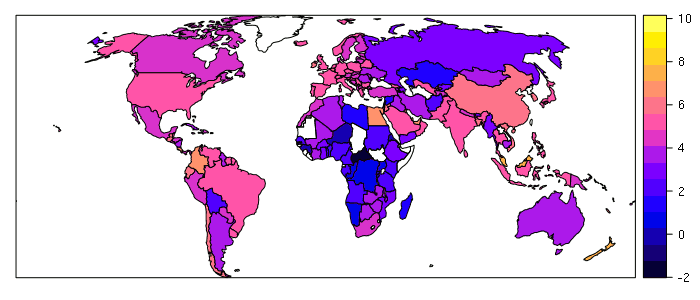# Importing and Visualizing World Bank Indicator Data

The World Bank aggregates a vast array of country-level data as indicators available from the World Bank Open Data portal. By joining that data with country polygons, you can create choropleth maps and perform geospatial analysis.

## Joining World Bank Data in ArcMap

1. The World Bank Open Data portal (0:00)
2. Joining with a country polygon shapefile (2:30)
3. Using the field calculator to conver text fields to numeric fields (4:35)
4. Symbolizing World Bank data (5:45)

## Importing World Bank Indicator Data Into R

1. Search for an indicator of interest on data.worldbank.org. The variable used in this example is Fertilizer consumption (kilograms per hectare of arable land)
3. Review the CSV file in a spreadsheet program to find the latest year with adequate data for your purposes
5. Modify the script below to join your World Bank data to the country polygons and save to a new shapefile:
```# Import attribute CSV file
# Note that the first four row of World Bank CSV files are
# metadata that can be skipped with the "skip=4" parameter.
# The year column names will have an X prepended to them
# (e.g. X2014) to avoid confusing the column names with numbers.

skip=4, stringsAsFactors=F)

# Import tract polygons
# The "dsn" parameter is the directory where the shapefile is located.
# A dot "." means the current directory.
# The "layer" parameter is the shapefile name (without the .shp suffix)

library(rgdal)

# Reproject the polygons to an cartographically-valid Robinson projection

robinson = CRS("+proj=robin +lon_0=0 +x_0=0 +y_0=0 +datum=WGS84 +units=m +no_defs")
countries = spTransform(countries, robinson)

# Merge (join) the attributes to the polygons.
# The World Bank country code in the polygon file is "WBA2016."

countries =  merge(countries, attributes, by.x = "WBA2016", by.y = "Country.Code")

# Convert the appropriate column to a new integer column.
# Data read from a CSV file may be characters rather than numbers
# and need to be converted to numbers using as.double().
# The name of this column wih vary depending on what variable(s) you use

countries\$FERTILIZER = as.double(countries\$X2014)

# Keep only needed columns

countries@data = countries@data[,c("NAME", "WBA2016", "FERTILIZER")]

# Write shapefile

writeOGR(countries, dsn=".", layer="fertilizer", driver="ESRI Shapefile", overwrite_layer=T)

# Optional diagnostic plot
# The log() transformation is needed because there are a handful
# of countries with very high fertilizer use that skew the distribution

library(sp)
countries\$FERTILIZER = log(countries\$FERTILIZER)
spplot(countries, zcol="FERTILIZER")
```2014 World Fertilizer Use (log kg per ha of arable land, 2017 FAO)